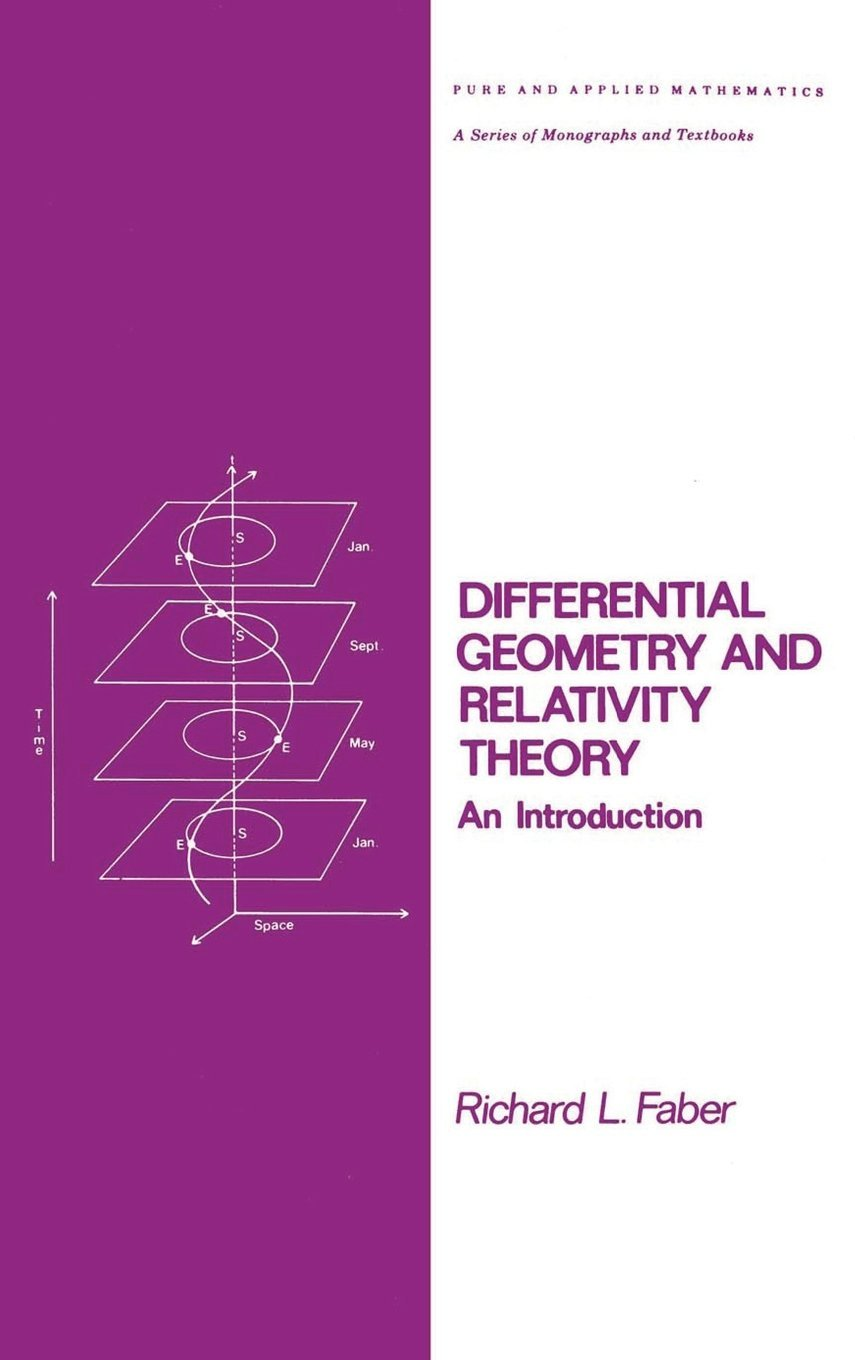Differential Geometry (and Relativity)
Classnotes from Differential Geometry and Relativity Theory, An Introduction by Richard L. Faber, Marcel Dekker (1983)Copies of the classnotes are on the internet in PDF and PostScript.

• Chapter 1: Introduction. PDF.
• Section 1-1: Curves. PDF.
• Section 1-2: Gauss Curvature. PDF.
• Section 1-3: Surfaces in E3. PDF.
• Section 1-4: First Fundamental Form. PDF.
• Section 1-5: Second Fundamental Form. PDF.
• Section 1-6: The Gauss Curvature in Detail. PDF.
• Section 1-7: Geodesics. PDF.
• Section 1-8: The Curvature Tensor and the Theorema Egregium. PDF.
• Section 1-9: Manifolds. PDF.
• Supplement. Equation Sheet for Chapter 1. PDF
• Supplement. Wald's General Relativity Notes webpage.
• Supplement. Hawking and Ellis's The Large Scale Structure of Space-Time Notes webpage.
• Chapter 2: Special Relativity: The Geometry of Flat Spacetime. PDF.
• Section 2-1: Inertial Frames of Reference. PDF.
• Section 2-2: The Michelson Morley Experiment. PDF.
• Section 2-3: The Postulates of Relativity. PDF.
• Section 2-4: Relativity of Simultaneity. PDF.
• Section 2-5: Coordinates. PDF.
• Section 2-6: Invariance of the Interval. PDF.
• Section 2-7: The Lorentz Transformation. PDF.
• Section 2-8: Spacetime Diagrams. PDF.
• Section 2-9: Lorentz Geometry. PDF.
• Section 2-10: The Twin Paradox. PDF.
• Section 2-11: Temporal order and Causality. PDF.
• Supplement. Das' The Special Theory of Relativity, A Mathematical Exposition, 1.1. Minkowski Vector Space V4. PDF.
• Supplement. Das' The Special Theory of Relativity, A Mathematical Exposition, 1.2. Lorentz Mappings of V4 (partial). PDF.
• Supplement. Das' The Special Theory of Relativity, A Mathematical Exposition, 1.3. The Minkowski Tensors (partial). PDF
• Supplement: Velocity and Acceleration in Polar Coordinates (This section of Calculus 3 notes includes proofs of Kepler's three laws of planetary motion.) PDF.
• Chapter 3: General Relativity: The Geometry of Curved Spacetime. PDF.
• Section 3-1: The Principle of Equivalence. PDF.
• Section 3-2: Gravity as Spacetime Curvature. PDF.
• Section 3-3: The Consequences of Einstein's Theory. PDF.
• Section 3-6: Geodesics. PDF.
• Section 3-7: The Field Equations. PDF.
• Section 3-8: The Schwarzschild Solution. PDF.
• Section 3-9: Orbits in General Relativity. PDF.
• Section 3-10: The Bending of Light. PDF.
• Supplement: Based on Foster and Nightingale's A Short Course in General Relativity. Black Holes. PDF.
Other Notes on Differential Geometry and Relativity